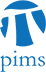## SCAIM Seminar: Multi-Level Monte Carlo Finite Element methods for elliptic PDEs with stochastic coefficients

• Date: 06/22/2010
Lecturer(s):
Andrea Barth (ETH Zurich)
Location:

University of British Columbia

Description:

It is a well-known property of Monte Carlo methods that quadrupling the sample size halves the error. In the case of simulations of a stochastic partial differential equations, this implies that the total work is the sample size times the discretization costs of the equation. This leads to a convergence rate which is impractical for many simulations, namely in finance, physics and geosciences. With the Multi-level Monte Carlo method introduced herein, the overall work can be reduced to that of the discretization of the equation, which results in the same convergence rate as for the standard Monte Carlo method. The model problem is an elliptic equation with stochastic coefficients. Multi-Level Monte Carlo errors and work estimates are given both for the mean of the solutions and for higher moments. Numerical examples complete the theoretical analysis.

Schedule:

12:30 - 2:00pm, WMAX 216.